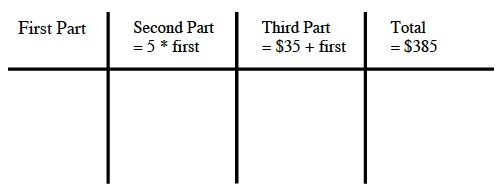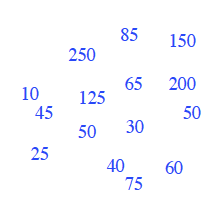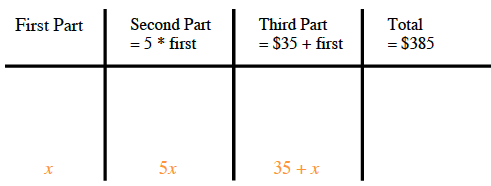### Home > AC > Chapter 3 > Lesson 3.1.1 > Problem3-7

3-7.

Ms. Nguyen needs to separate $\385$ into three parts to pay some debts. The second part must be five times as large as the first part. The third part must be $\35$ more than the first part. How much money must be in each part?

Make a table and try various amounts.

Write the blue numbers at the right into the table to find how much money must be in the first part, second part, and third part so that the total is $\385$.

Use the expressions shown in the table above and your trials in the table to write an equation. Solve your equation. Does your answer make sense?